# Modelling Colinearity in the Presence of Non–Normal Error: A Robust Regression Approach

Afeez Mayowa BABALOLA*1 and Maxwell Obubu2

1Department of Statistics, University of Ilorin, Nigeria

2Department of Statistics, Nnamdi Azikwe University, Nigeria

Received Date: July 15, 2019  Published Date: July 26, 2019

#### Abstract

Multicollinearity and non-normal errors, which lead to unwanted effect on the least square estimator, are common problems in multiple regression models. It would therefore seem important to combine estimation techniques for addressing these problems. In the presence of multicollinearity and non-normal errors, different estimation techniques were examined, namely, the Ordinary Least Squares (OLS), Ridge Regression (R), Weighted Ridge regression (WR), Robust M-estimation (M), and Robust Ridge Regression with emphasis on M-estimation (RM). When compared with the condition of Collinearity, the results of a simulated study shows that Robust Ridge (RM) provides a more efficient estimate then the other estimators considered. When both Collinearity and non-normal errors were considered, the M-estimators (M) produces a more efficient and precise estimates.

Keywords: Multicollinearity; Ordinary least squares (OLS); Regressor; Simulation; M-estimator; Estimates

#### Introduction

Regression analysis is “robust” in that it will typically provide estimates that are reasonably unbiased and efficient even when one or more of the assumptions is not completely met. However, a large violation of one or more assumptions will result in poor estimates and, consequently, the wrong conclusions being drawn. Two important problems are considered in regression analysis: multicollinearity and non–normal error distribution. Multicollinearity is the term used to describe cases in which the regressors are correlated among themselves. Another common problem in regression estimation methods is that of non–normal errors. The term simply means that the error distributions have fatter tails than the normal distribution. These fat–tailed distributions are more prone than the normal distribution to produce outliers, or extreme observations in the data. The Ordinary Least Squares estimators (LS) of coefficients are known to possess certain optimal properties when explanatory variables are not correlated among themselves, and the disturbances of the regression equation are independently, identically distributed normal random variables. This study focuses on violation of the assumption that there is no linear relationship between the explanatory variables and the disturbances distribution is non–normal. If this assumption is violated, multicollinearity ensues which leads to inflation of Standard Error (SE) of the coefficients of the estimated parameters [1-6]. Multicollinearity is a case of multiple regression in which the predictor variables are themselves highly correlated, meaning that one can be linearly predicted from the others with a non–trivial degree of accuracy. If there is no linear relationship between the regressors, they are said to be orthogonal. When the regressors are orthogonal, the inferences such as;

(i) identifying the relative effects of the regressor variables,

(ii) prediction and/or estimation, and

(iii) selection of an appropriate set of variables for the model

Can be made easily. Unfortunately, in most applications of regression, the regressors are not orthogonal. Sometimes the lack of orthogonality is not serious. However, in some situations the regressors are nearly perfectly linearly related, and in such case the inferences based on the regression model can be misleading or erroneous. When there are near–linear dependencies among the regressors, the problem of multicollinearity is said to exist. Non–normal error is a situation whereby the errors have fatter tails than the normal distribution tail. The Gauss-Markov Theorem says that OLS estimates for coefficients are BLUE when the errors are normal and homoscedastic. When errors are nonnormal, the ‘E’ property (Efficient) no longer holds for the estimators and in small samples, and the standard errors will be biased. When errors are heteroscedastic, the standard errors become biased. Thus, we typically examine the distribution of the errors to determine whether they are normal.

##### M – Estimation

The theory of robustness developed by Huber and Hampel (1960) laid the foundation for finding practical solutions too many problems, when statistical concepts were vague to serve the purpose. Robust regression analyses have been developed as an improvement to least squares estimation in the presence of outliers and to provide us information about what a valid observation is and whether this should be thrown. The primary purpose of robust regression analysis is to fit a model which represents the information in the majority of the data. Robust regression is an important tool for analyzing data that are contaminated with outliers. It can be used to detect outliers and to provide resistant results in the presence of outliers. Many methods have been developed for these problems. Many researchers have worked in this field and described the methods of robust estimators. The class of robust estimators includes M-, L- and R-estimators. The M-estimators are most flexible ones, and they generalize straightforwardly to multiparameter problems, even though they are not automatically scale invariant and have to be supplemented for practical applications by an auxiliary estimate of scale any estimate [6-10].

##### Motivation

This research work was motivated by the idea that in the presence of substantial collinearity and non–normal error in a regression model, the results obtained from Ordinary Least Square Estimator are unreliable. The effect and remedy to such influences needs to be study.

#### Material and Methods

The basic requirement of the LS estimation of a linear regression is that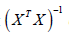exits. The two major reasons that the inverse does not exit is (1) when > n , (2) multicollinearity. When multicollinearity exits the design matrix is “ill conditioned” and invertible. A simple way to guarantee the invertibility is adding a constant to the diagonal matrix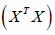before estimating the coefficients. Hoerl and Kennard (1970) proposed the ridge estimatorAnother way of understanding the ridge estimator is; recall that the LS estimator2 tends to minimizes the residual sum square i.e.but when collinearity exist then ˆβ is sensitive to small change in the data set and we uses ridge estimator that penalizes the value of β by minimizing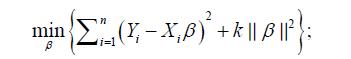The first term of equation (3.1.5) is regarded as the loss and the second term of the equation is the penalty. Note that: with larger k the penalty of β becomes stronger [10-14].

##### Robust regression methods

Robust Regression estimators have been proven to be more reliable and efficient than Least Squares Estimator especially when disturbances are non-normal. “Non-normal disturbances” are disturbances distribution that have heavy and fatter tails than the normal distribution and are prone to produce outliers. Since outliers greatly influence the estimated coefficients, standard errors and test statistics, the usual statistical procedure may be most inefficient as the precision of the estimator has been affected. Several different classifications of robust regression exist. Two of the most commonly considered group are: L-estimators and M-estimators. The L-estimators is the earliest one. One important member of regression L-estimators is called Weighted Least Squares estimator (WLS).

##### Weighted Least Squares Estimator

Given a regression model: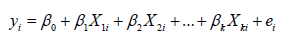where are random errors that is independent and identically distributed with mean zero and variance . Suppose we have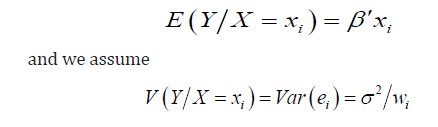whereare known positive numbers. The model can now be re-written asThe Weighted Least Squares estimator also known as the Generalized Least Squares estimator is given by: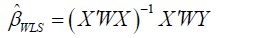where W is a diagonal matrix with diagonal elements. The diagonal elements of W matrix are set equal to:##### M - Estimation

The linear model: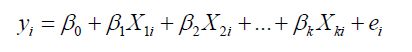This can also be written in matrix form as:

The general M-estimator minimizes the objective function

##### Robust ridge regression estimates

The following formula is used to compute robust ridge estimates:

wheredenotes the coefficient estimates from the robust estimators. Many methods of selecting appropriate k values have been proposed in the literature. In this study, the method proposed by Hoerl, Kennard and Baldwin (HKB) (1975), based on Least Squares estimator, has been used for the selection of the k values, building on robust estimators:

where p is the number of regressors andis the robust scale estimator.

##### Weighted ridge estimator (Wr)

Weighted Ridge Estimatorcan be computed using the following formula:

βW denotes the coefficient estimates from the Weighted Least Square estimators.

##### Ridge M-Estimator (Rm)

The Ridge M-estimator of the parameter β is given by:where the M-estimator procedure of is used rather than Least Square estimator in computing and values in order to reduce the effect of non-normality on the value choosen for , and the value can be written as: [16-18]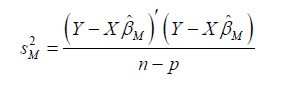#### Results and Discussion

##### Data simulation

The data utilized for this work were simulated from R (www. cran.r-project.org) statistical package. The three predictor variables are generated form the multivariate normal package as follows:is the vector of the true population value that the data set is simulated from.the specified correlation and it varies between Tolerable Non-Orthogonal Correlation Point and the extreme correlation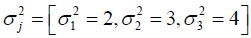is the variance vector of the predictors.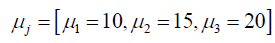is the specified mean vector of the predictors.

Sigma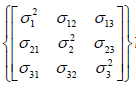Variance cov ariance matrixOne important factor in this study is the disturbances distribution. Therefore, the residual term was simulated from two distributions, namely:

• The univariate normal distribution with mean 0 and standard deviation σ =10, and

• Cauchy distribution with median zero and scale parameter one

e1 = rnorm(n ∗ p,0,σ )

e2 = rcauchy (n ∗ (1− p),0,1)

e = c (e1,e2)

Where p represents the proportion of error from the normal distribution.

Y = Xβ +ε

And the response is simulated with the relationship given below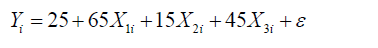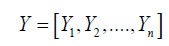is the vector of the generated dependent variable.

##### Correlation structure

For the TNCP (tolerable non orthogonal correlation)For the severe correlationIn order to compare the performance of the estimators, the Mean Square Error (MSE) was computed for the data set at various sample sizes and at different iteration. In each case, the average MSEs for each sample were reported. The true parameter values were compared with the regression estimates at different sample sizes (Table 1).

Table 1: Summary of Estimate of Coefficients and Standard Error at Various Sample Size for Tolerable Non-Orthogonal Correlation.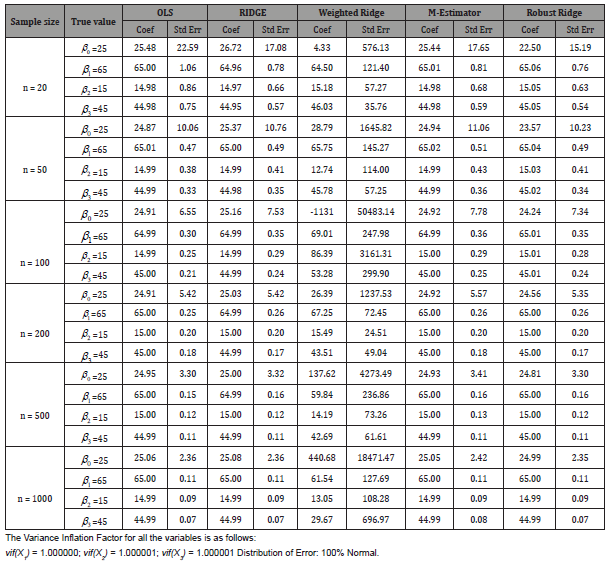From Table 1 it is observed that the value of estimated regression coefficients for all the estimators considered is an unbiased estimate of the population parameter when the regressors are orthogonal, and the disturbances of the regression equation are independent, identically distributed normal random variables, except for Weighted Ridge which is over estimating the parameter (Table 2).

Table 2: Mean Square Error of the estimators at various sample sizes.From Table 2, it is observed that the MSEs asymptotically tend to zero as the sample sizes become large, which is indicating the consistency of the estimators except that of the Weighted Ridge which is not stable (Table 3).

Table 3: Summary of Estimate of Coefficients and Standard Error At Various Sample Size for Severe Correlation Level.From Table 3, it is observed that the result obtained from Weighted Ridge is inconsistent with true parameter and result obtained from other estimators considered in this study. It is also observed that as the sample sizes become large, the result obtained from other estimators except Weighted Ridge converges to the true parameter (Table 4).

Table 4: Mean Square Error of the estimators at various sample sizes.From Table 4, it is observed that the MSEs asymptotically tend to zero as the sample sizes become large, which is indicating the consistency of the estimators except that of the Weighted Ridge which is not stable. Where the MSE of Robust Ridge clearly show that it is more efficient than other estimator considered (Figure 1).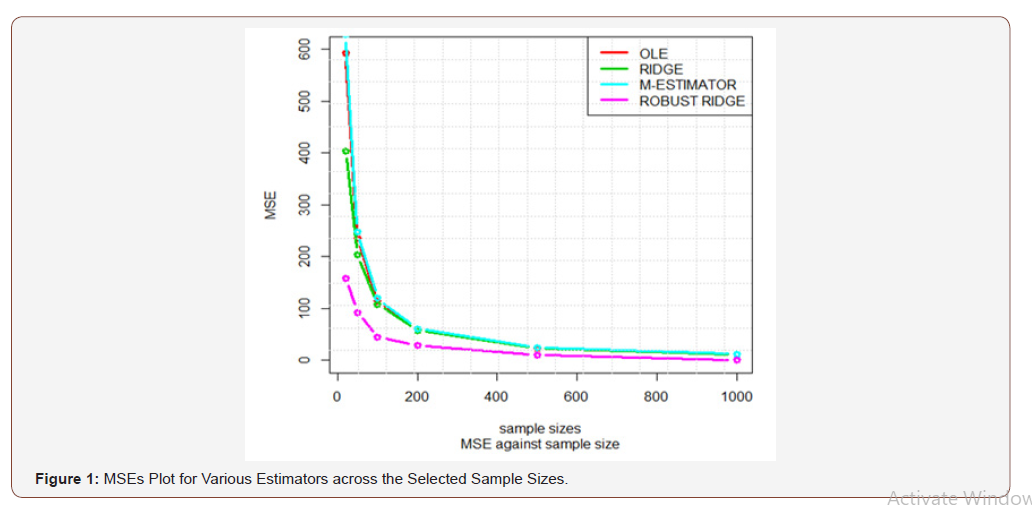From the plot above, it is observed that the MSE asymptotically tend to zero as the sample sizes becomes large, which indicate the consistency of the estimators (Table 5).

Table 5: Summary of Estimate of Coefficients and Standard Error At Various Sample Size for Tolerable Non-Orthogonal Correlation.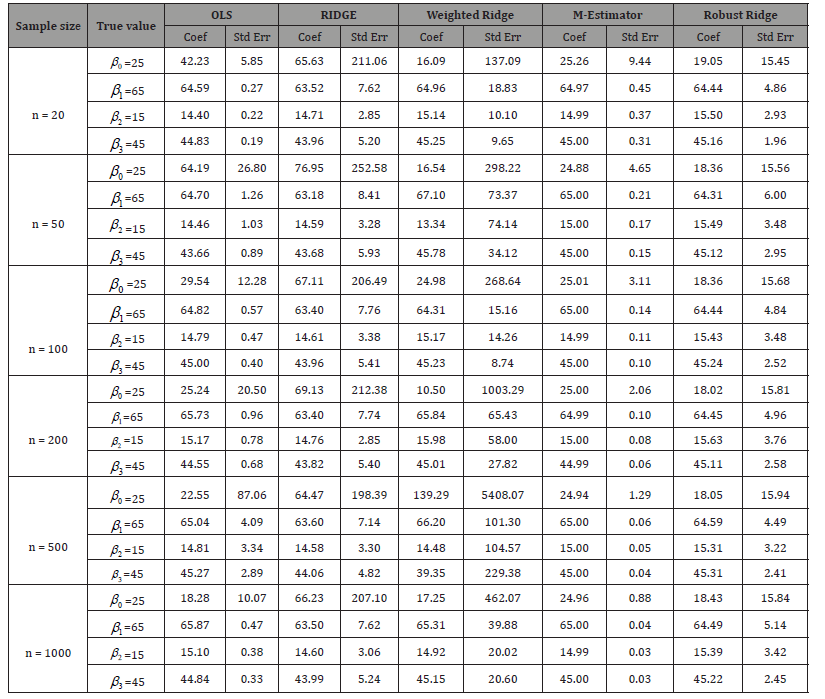From the above table, it is observed that the result obtained from Robust M-estimation is an unbiased estimate of the true parameter, while result obtained from other estimators considered in this study are biased which is as a result of non-normal error (Table 6).

Table 6: Mean Square Error of the estimators at various sample sizes.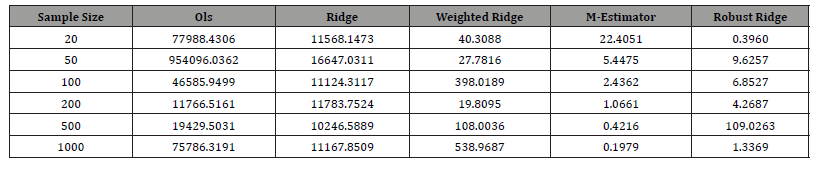From the Table above, it is observed that the MSE of M-estimation asymptotically tend to zero as the sample sizes become large, which is indicating the consistency of the estimator compared to other estimators considered in this study, while the result of MSE based on OLS is the worst among the estimators. Robust M-estimator is more efficient than other estimator considered (Table 7).

Table 7: Summary of Estimate of Coefficients and Standard Error at Various Sample Size for Severe Correlation Level.From the above table, it is observed that the result obtained from Robust M-estimation is an unbiased estimate of the true parameter, while result obtained from other estimators considered in this study are biased which is as a result of effect of collinearity and non-normal error (Table 8).

Table 8: Mean Square Error of the estimators at various sample sizes.From the table above, it is observed that the MSE of M-estimation asymptotically tend to zero as the sample sizes become large, which is indicating the consistency of the estimator compared to other estimators considered in this study, while the result of MSE based on OLS is the worst among the estimators. Robust M-estimator is more efficient than other estimator considered (Figure 2).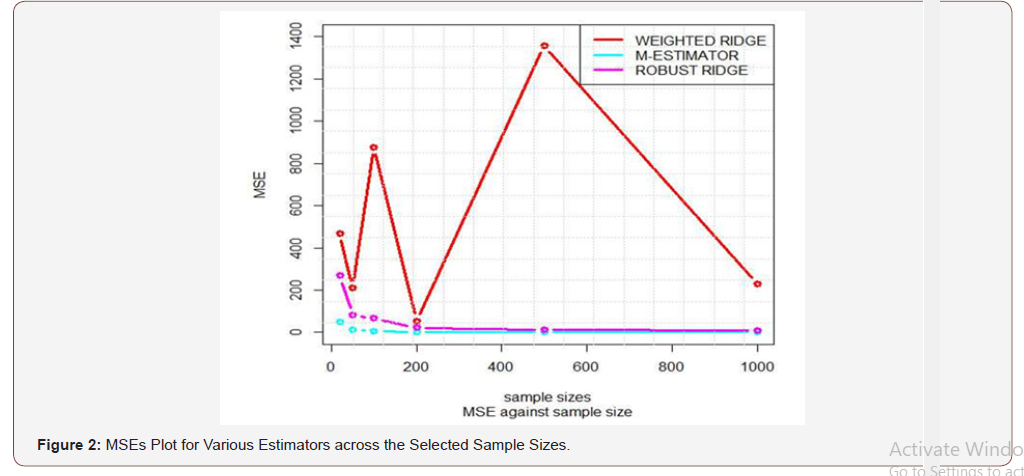#### Conclusion

From the simulation studies it can be concluded to a reasonable extend that In the presence of both multicollinearity and nonnormal error the OLS estimators produces relatively large Mean Squares Errors, hence it gives unstable parameter estimates. the sample size is inversely proportional to the mean square error of estimate i.e. the larger the sample size, the lower the mean square error and vice versa. To make any reasonable predictions and inferences in any multiple linear regressions, one must be certain that the predictors are non-orthogonal and the percentages of data that are outliers are minima before the commencement of the analysis. This study reveal that the consequence of collinearity in the presence of non-normal error is more severe compared to when the error distribution is normal. Finally, the results of comparisons shows that for the condition of collinearity, Robust Ridge estimates are more efficient than the other estimators considered. While for the condition of collinearity and non-normal error M-estimator produces estimates that were more efficient and precise.

#### Recommendation

This research work will help present and future researchers in choosing or determining the appropriate estimator for estimating the regression parameters when the traditional method for estimating the parameters is unreliable in the presence of both multicollinearity and non–normal error problem in a data set.

None.

#### Conflict of Interest

No conflict of interest

Article Details
Citation
Keywords
Scroll to
Scroll to Top